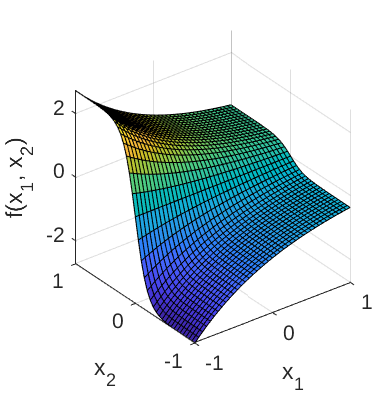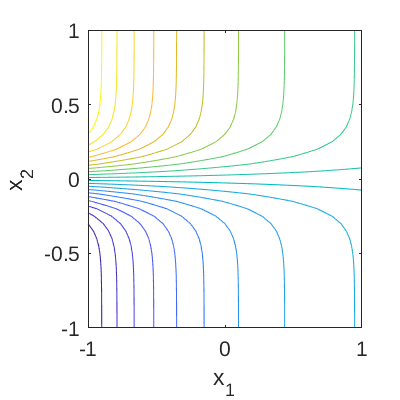# Exp-Tanh Function

In Owen et al. (2017), the Exp-Tanh function is used to test metamodeling approaches, namely polynomial chaos expansions and Gaussian process modeling.

## Description

The Exp-Tanh function is defined as:

f(\mathbf{x}) = \exp{(-x_1)} \tanh{(5x_2)},\;\;\; x_1, x_2 \in [-1,1],

where \mathbf{x} = \{x_1, x_2\} are the input variables.

Figure 1 and 2 show the surface and contour plots of the Exp-Tanh function, respectively.Figure 1: Surface plot of the Exp-Tanh function.Figure 2: Contour plot of the Exp-Tanh function.

## Inputs

For computer experiment purposes, the inputs x_1, x_2 are modeled as two independent uniform random variables.

No Variable Distribution Parameters
1 x_1 Uniform x_{1,\min} = -1, x_{1,\max} = 1
2 x_2 Uniform x_{2,\min} = -1, x_{2,\max} = 1

## Resources

The vectorized implementation of the Exp-Tanh function in MATLAB as well as the script file with the model and probabilistic inputs definitions for the function in UQLAB can be downloaded below:

uq_expTanh.zip (2.1 KB)

The contents of the file are:

Filename Description
uq_expTanh.m vectorized implementation of the Exp-Tanh function
uq_Example_expTanh.m definitions for the model and probabilistic inputs in UQLab
LICENSE license for the function (BSD 3-Clause)

## References

• N. E. Owen, P. Challenor, P. P. Menon, and S. Bennani, “Comparison of surrogate-based uncertainty quantification methods for computationally expensive simulators,” SIAM/ASA Journal on Uncertainty Quantification, vol. 5, no. 1, pp. 403–435, 2017. DOI:10.1137/15M1046812
1 Like